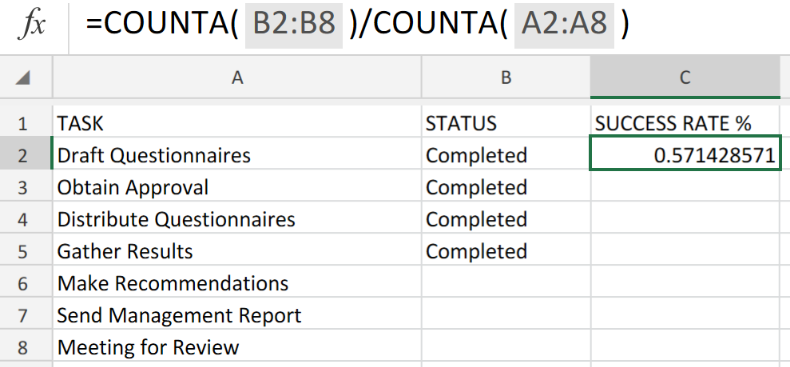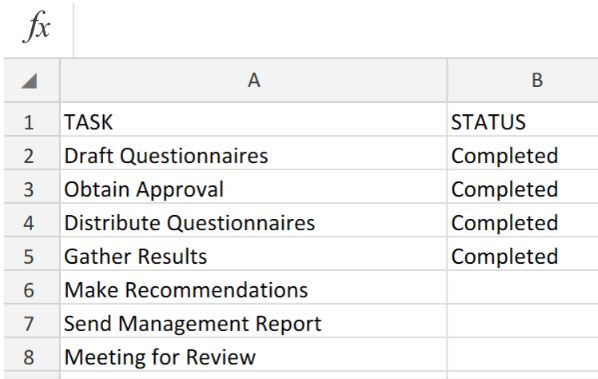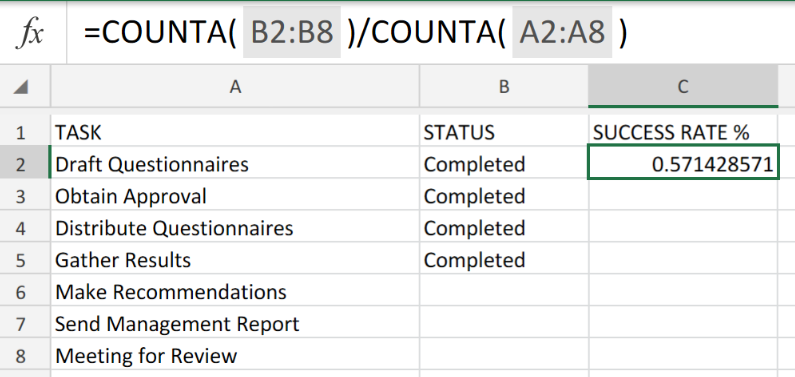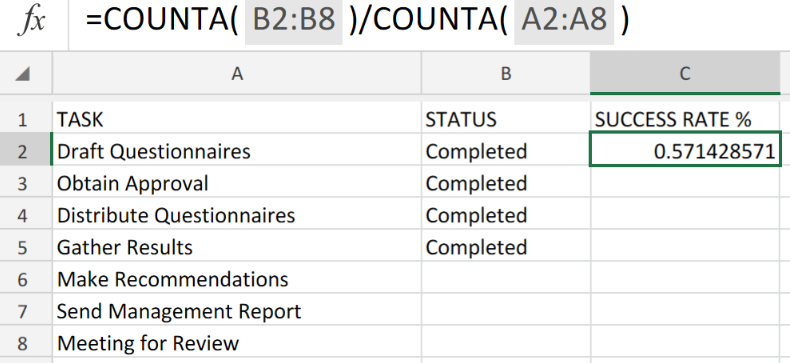Get instant live expert help with Excel or Google Sheets“My Excelchat expert helped me in less than 20 minutes, saving me what would have been 5 hours of work!”

#### Post your problem and you'll get expert help in seconds

Your message must be at least 40 characters
Our professional experts are available now. Your privacy is guaranteed.

# How to Calculate a Project Complete Percentage in ExcelFigure 1. of Percentage of Project Completion in Excel.

To determine the Percentage Completion Rate for any project consisting of a number of tasks, we can utilize the operation syntax of the COUNTA Function in Excel. The COUNTA Function operates by simply dividing completed tasks by the total amount of tasks.

## Generic Formula.

`=COUNTA(range1)/COUNTA(range2)`

## How to use the Excel COUNTA Function.

To determine the Percentage Completion Rate for a project having a set of tasks, we are going to utilize a simple formula syntax that is based on the Excel COUNTA Function.

• Arrange items identified as the tasks to be completed in our Excel sheet. They should be arranged in stages starting from the first to the last task.Figure 2. of List of Completed Tasks.

In the example illustrated above, the total number of identified tasks are arranged in the first column A. Those labeled as Completed in the second column B are those tasks that have already been carried out.

1. We will then proceed to label a third column C, as Success Rate. This is where the calculated Project Completion Percentage will be located after calculation.

We can achieve this by entering the following formula syntax derived from the COUNTA Function, directly into the formula bar of cell C2 of our worksheet example;

`=COUNTA(B2:B8)/COUNTA(A2:A8)`Figure 3. of Percentage Completion Rate Function in Excel.

We count Completed Tasks by counting non-blank cells located within the cell range B2:B8 of our worksheet example. We count Total Tasks by counting non-blank cells located within the cell range B2:B8 of our worksheet example. The Excel COUNTA Function will by default, count cells in our worksheet that include text or numbers.Figure 4. of Final Result.

## Instant Connection to an Expert through our Excelchat Service

Our live Excelchat Service is here for you. We have Excel Experts available 24/7 to answer any Excel questions you may have. Guaranteed connection within 30 seconds and a customized solution for you within 20 minutes.

### Did this post not answer your question? Get a solution from connecting with the expert.Another blog reader asked this question today on Excelchat:## Subscribe to Excelchat.coAnother blog reader asked this question today on Excelchat: